Navigation
Home Page

# Maths & Problem Solving

## Expanded method for subtractionIn this clip we explore how place value counters can be used to support children's understanding of subtraction.

## Chunking method for long divisionIn this clip we explore how chunking can used when dividing larger numbers.

Build it up!!

We start with any four numbers (not zero!)We then add in pairs and place the totals above them :-And we then add in pairs the new numbers we just got.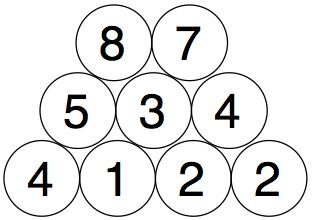We do the same with those two numbers to get our final number.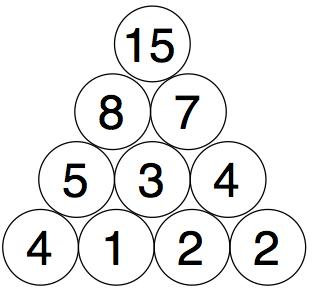You need to find four starting numbers to place at the bottom so that when you get to the top it's 15 each time.

Try to find as many starting four numbers as you can.

Secret Number

##### Stage: 1This is a game for two players and a simple calculator.
Annie and Ben are playing. Annie puts her secret number into the calculator without showing Ben.
Annie then asks Ben, "What do you want to add?"
Ben tells Annie the number he wants to add. "I want to add four."
Annie presses the 'add' button and then the four button. The calculator now shows '4'. Annie gives the calculator to Ben.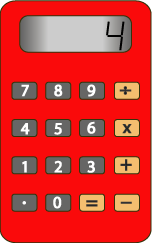Ben presses the 'equals' button and the calculator gives the answer '10'.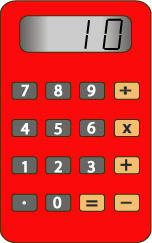What was Annie's secret number?
How do you know?
You could play this with a friend. If you work out your friend's secret number correctly, it is your turn to put in a secret number of your own. You could score a point for every one you get right.

A multiplication version of the game might go like this:
Charlie puts in a secret number and asks Dana, "What do you want to multiply it by?"
Dana replies, "Multiply it by 5."
Charlie puts in 'times' and '5' and hands the calculator to Dana.
When Dana presses the 'equals' button the calculator shows '35'.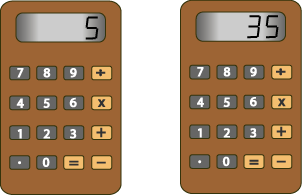Dana now has to work out Charlie's secret number. What was it?
How do you know?

Try playing this version with your friend too!
Pass the peas please!

This is a game for two or more players.

Materials needed:

• Plastic cup
• Dried peas
• Game board
• Paper and pencil each for calculating and recording scoresEach player begins by writing 50 on their score sheet.
Place two dried peas into a plastic cup.
Toss the peas onto the game board.
Add the two numbers the peas land on.
Take the sum away from 50 and record the remainder.
Next player takes a turn.
In the following turns, the players take the sum of the numbers away from the new remaining number.
The first player to reach zero wins.

Change the game by:
Using three peas instead of two.
Altering the starting number from 50 to 100.
Multiplying the numbers that the peas land on.
Making a different size game board with a different set of numbers.

Part the Polygons

Using three straight lines divide this rectangle into four parts.
Each part must contain a triangle, a rectangle, a pentagon, a hexagon, a cylinder and an oval.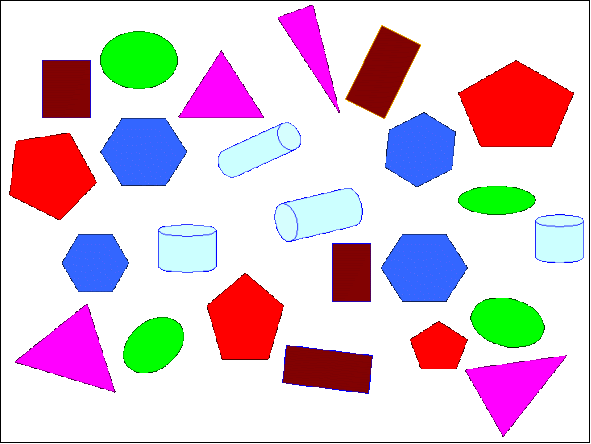Is a Square a Rectangle?

How many rectangles can you find in this shape?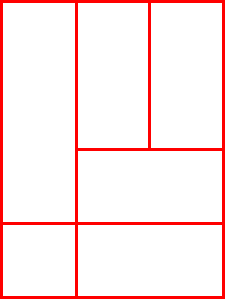How many of the rectangles are differently shaped?

Can you find any that are 'similar' - that is the same shape but a different size?

Target challenge!

You have two sets of the digits from 0 to 9.Arrange FOUR of these digits in each  box to make a four-digit number as close to the target number as possible.  If you find that easy, try again but this time you can ONLY use each digit ONCE- cross them out as you go so you don't use a digit too many times!!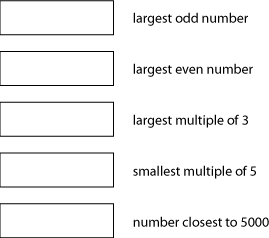## Maths expectations for each year group

• St George's Church of England Primary Academy, Admiralty Street, Stonehouse, Plymouth, Devon, PL1 3RX
• T: 01752 224863
• E: admin@stgeorgesstcmat.org
Top